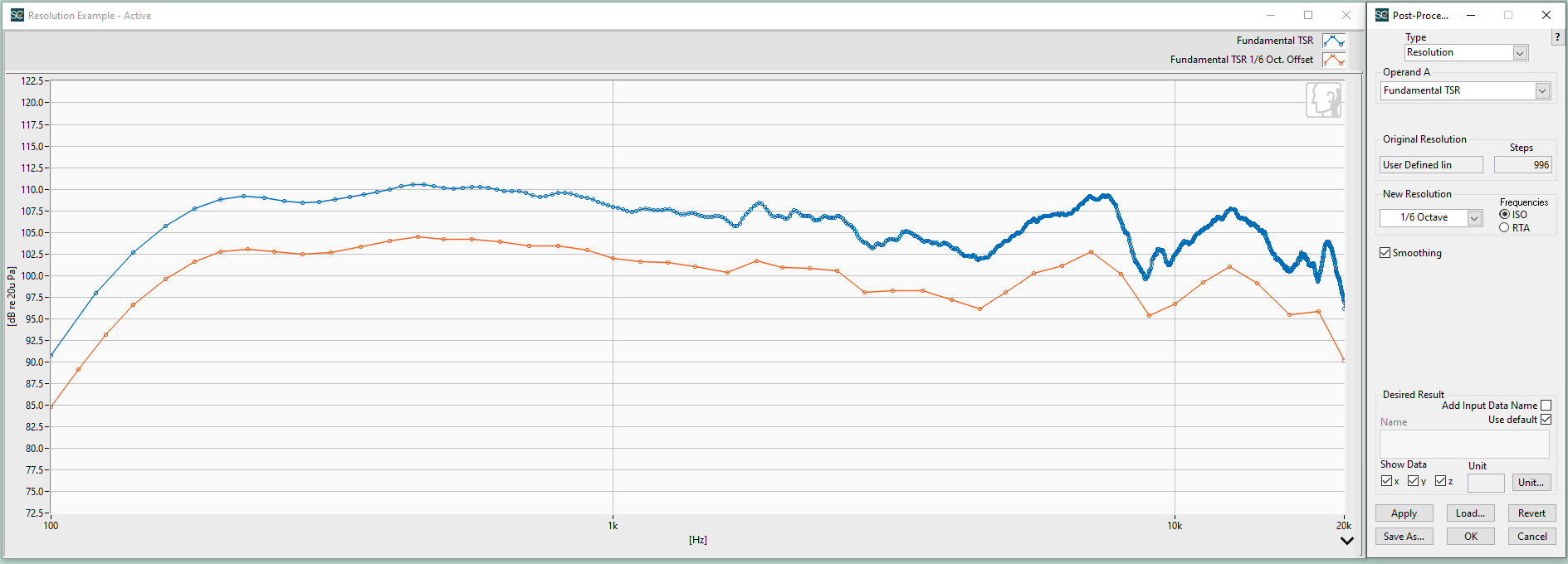# Can I change the resolution of a curve once it has been output from Analysis?

Yes! The Resolution post processing step was developed to perform this specific function.

Using this step, a curve can be resampled to a new resolution. e.g., a linear spectrum can be synthesized to 1/3 octave or a 1/24 octave curve can be power summed to 1/12 octave. It is intended for use with curves created by the HarmonicTrak, Heterodyne and Time Selective Response algorithms.

A smoothing function is also included in the Resolution step as follows:

• The smoothing function is applied before resampling
• The smoothing width is the same as the selected resolution

With Smoothing disabled, the Resolution step creates the output curve by linearly interpolating the magnitude and phase data of Operand A at frequencies defined by the selected resolution. With Smoothing enabled, the Resolution step will first linearly interpolate Operand A data to the highest possible resolution. (This is the equivalent of the original curve being “User defined lin” with a value of 1 Hz). It then resamples the interpolated data at the selected resolution to create the resulting curve in the Memory List.

Smoothing is generally only useful if the final curve resolution is higher than or “not a mathematical factor” of Operand A’s resolution. For example, Operand A is non-standard 1/10th octave resolution and the selected result resolution is standard R40 1/12th octave. In this case, smoothing should applied. Resolution will interpolate values for basically every frequency between the original 1/10th octave frequencies. Then, just the values corresponding to 1/12th octave frequencies will be picked out to create the resulting curve in the Memory List.

Another option available in the Resolution step is to output the resulting curve with ISO or RTA frequencies.  In measurement resolutions above 1/3rd octave, ISO and RTA frequency points no longer coincide. When the “New Resolution” field is set to 1/6 octave or above, you can select either ISO or RTA frequencies. This is useful for comparing measurements made with HarmonicTrak (ISO frequency points) to measurements made with the RTA virtual instrument or analysis step.20 Hz linear resolution (blue curve) changed to 1/6 octave resolution and offset by 6 dB (orange curve)